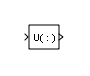# Reshape

Change dimensionality of signal

• Library:

HDL Coder / Math Operations

•## Description

The Reshape block changes the dimensionality of the input signal to a dimensionality that you specify, using the Output dimensionality parameter. For example, you can use the block to change an N-element vector to a 1-by-N or N-by-1 matrix signal.

## Ports

### Input

expand all

Input signal whose dimensions are changed based on the Output dimensionality parameter.

Data Types: `half` | `single` | `double` | `int8` | `int16` | `int32` | `int64` | `uint8` | `uint16` | `uint32` | `uint64` | `Boolean` | `fixed point` | `enumerated` | `bus`

### Output

expand all

Output signal created with the dimensions specified in the Output dimensionality parameter.

Data Types: `half` | `single` | `double` | `int8` | `int16` | `int32` | `int64` | `uint8` | `uint16` | `uint32` | `uint64` | `Boolean` | `fixed point` | `enumerated` | `bus`

## Parameters

expand all

Specify the dimensionality of the output signal.

Output DimensionalityDescription

1-D array

Converts a multidimensional array to a vector (1-D array) array signal. The output vector consists of the first column of the input matrix followed by the second column, and so on. (This option leaves a vector input unchanged.)

Column vector

Converts a vector, matrix, or multidimensional input signal to a column matrix, a M-by-1 matrix, where M is the number of elements in the input signal. For matrices, the conversion is done in column-major order. For multidimensional arrays, the conversion is done along the first dimension.

Row vector

Converts a vector, matrix, or multidimensional input signal to a row matrix, a 1-by-N matrix where N is the number of elements in the input signal. For matrices, the conversion is done in column-major order. For multidimensional arrays, the conversion is done along the first dimension.

Customize

Converts the input signal to an output signal whose dimensions you specify, using the Output dimensions parameter.

Derive from reference input port

Creates a second input port, Ref, on the block. Derives the dimensions of the output signal from the dimensions of the signal input to the Ref input port. Selecting this option disables the Output dimensions parameter. When you select this parameter, the input signals for both inport ports, U and Ref, must have the same sampling mode (sample-based or frame-based).

#### Programmatic Use

 Block Parameter: `OutputDimensionality` Type: character vector Value: ```'1-D array'``` | `'Column vector (2-D)'` | `'Row vector (2-D)'` | `'Customize'` | ```'Derive from reference input port'``` Default: ```'1-D array'```

Specify the dimensions for the output signal. The value can be a one- or multi-element vector. A value of `[N]` outputs a vector of size `N`. A value of `[M N]` outputs an `M`-by-`N` matrix. The number of elements of the input signal must match the number of elements specified by the Output dimensions parameter. For multidimensional arrays, the conversion is done along the first dimension.

#### Dependency

To enable this parameter, set Output dimensionality to `Customize`.

#### Programmatic Use

 Block Parameter: `OutputDimensions` Type: character vector Value: `'[integer,intger]'` | Default: `'[1,1]'`

## Block Characteristics

 Data Types `Boolean` | `bus` | `double` | `enumerated` | `fixed point` | `half` | `integer` | `single` Direct Feedthrough `yes` Multidimensional Signals `yes` Variable-Size Signals `yes` Zero-Crossing Detection `no`